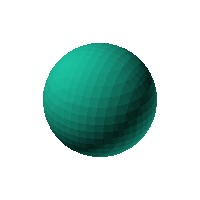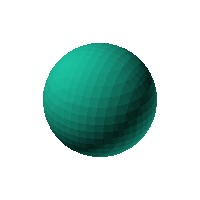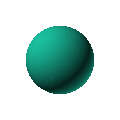Blob Farm# blob37The blob in all its glory:The ur-blob: a simple sphere.

 Click on the snapshot to download the blob's stl file.Octave Code:
```  # name of the blob
project = "blob37";

function w = f(x2,y2,z2,c,r,e)

x  = (x2-c(1))/r(1);
y  = (y2-c(2))/r(2);
z  = (z2-c(3))/r(3);
# function at origin must be <0, and >0 far enough away.  w=0 defines the surface

w = x.^2+y.^2+z.^2-1;

endfunction;

c_outer = [0,0,0];
r_outer = [30,30,30];

source("../octave/func2stl_v01.m");  # do all the calculations
```
GNU Octave